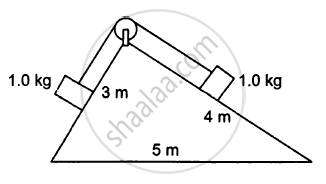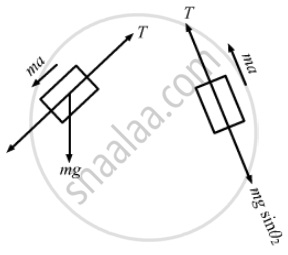# Consider the Situation Shown in Figure (5−E9). All the Surfaces Are Frictionless and the String and the Pulley Are Light. Find the Magnitude of Acceleration of the Two Blocks. - Physics

Sum

Consider the situation shown in the following figure  All the surfaces are frictionless and the string and the pulley are light. Find the magnitude of acceleration of the two blocks.#### Solution

Mass of each block is 1 kg, $\sin \theta_1 = \frac{4}{5}$
$\sin \theta_2 = \frac{3}{5}$
The free-body diagrams for both the boxes are shown below:mgsinθ1 − T = ma    ...(i)
T − mgsinθ2 = ma    ...(ii)
Adding equations (i) and (ii),we get:
mg(sinθ1 − sinθ2) = 2ma
⇒ 2a = g (sinθ1 − sinθ2)
$\Rightarrow a = \frac{g}{5} \times \frac{1}{2}$
$= \frac{g}{10}$

Concept: Newton’s Second Law of Motion
Is there an error in this question or solution?

#### APPEARS IN

HC Verma Class 11, Class 12 Concepts of Physics Vol. 1
Chapter 5 Newton's Laws of Motion
Q 25 | Page 81